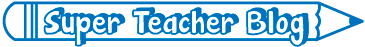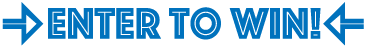# What's New on STW

We add new printable worksheets, games, and teaching tools all the time! This page highlights some of our most recent additions to the site.

1,283 new files added over the past 365 days.Multiply length by width to find area. Add or multiply fractions with like denominators. Solve multi-step word problems at a 4th grade level.
Multiply 3-digit numbers by a single-digit. Convert feet to yards. Divide with A 3-digit dividend and a 1-digit divisor.
Read, analyze, and interpret data on a table. Solve by using operations with mixed numbers. Division of a fraction by a whole number. Add fractions with like denominators.
This set of 5 worksheets has a variety of single and multi-step problems. Skills involve operations with fractions and division.
Subtract decimals (money), add fractions with like denominators, calculate perimeter, and calculate weight.
Calculate both the perimeter and area of these parallelograms. Side and height lengths include decimals and fractions.
On this worksheet, your students will find both the perimeter and area of the shapes.
Find the area and perimeter of each of the nine parallelograms given.
Students can use crayons or colored pencils to finish this map of Washington DC.
This is a color map of the nation's capital city. Several landmarks are included, as well as the Potomac River and bordering states.Have you seen the Super Teacher Blog? We post fun, new teaching ideas every month.
Be sure to check it out. Go To STW's Blog
This worksheet has three parts. In part one, students find the areas of three triangles. In part two, they find the areas of a triangle that is divided into parts. In part three, they are given the area and they calculate the height.
In the top section, your pupils will find the area of three triangles. In the middle section, find the areas of triangle parts. In the final section, they are given the area and must calculate the height.
First, find the area of three different triangles. Then find the areas of triangles within a larger shape. Then find the height when you are given the area.
This worksheets has 9 trapezoid models. Students can practice finding the area of each one.
Use the formula shown at the top to calculate the areas of the 9 trapezoids pictured.We give away a different prize for teachers each month.
Enter to win on our contest page! Go to STW's Contest Page
Use the formula A=bh to find the areas of the 9 parallelograms shown.
Here's a worksheet with 9 parallelogram pictures. Calculate the area of each by multiplying base times height.
This page has nine parallelogram illustrations. Multiply the base times the height to find the area of each.
This map of New Brunswick includes many important landmarks, major cities, and bodies of water.
This is a full-colour version of our Newfoundland and Labrador map.
This map includes important landmarks in Newfoundland and Labrador.
¿Habla español?
Many of our worksheets have been translated into Spanish! Click thebutton below worksheet descriptions to view our Spanish translations. Learn more.
Work on fixing grammar, spelling, and other writing mistakes with these next five worksheets.
This next set of Fix the Sentences worksheets will encourage students to continue to perfect their proofreading skills.
Continue practicing proofreading skills with this next set of daily Fix the Sentences worksheets.
This week students will continue finding spelling, punctuation, and other errors in these grade-appropriate proofreading sentences.
Operations with money, measurement, and elapsed time are some of the skills students will work on with this week's daily math problems.
This set of daily word problems includes deciphering a graph, interpreting data on a table, and operations problems.
This next set of daily word problems includes several multi-step operations problems and an elapsed time problem.
This set of five daily word problems includes multi-step operations problems, elapsed time, and more!
For each triangle shown, students calculate the perimeter and the area.
Find the perimeter and the area of each triangle shown. This advanced-level worksheet has 3-digit decimal numbers.
Calculate the areas of the triangles on these task cards. Use these cards for exit slips, classroom projects, or on your whiteboard document camera.
Print these area of a triangle task cards. Use them for classroom games, exit slips, on your document camera, or scavenger hunts.
This file contains 30 area of a triangle task cards. Use them for classroom scavenger hunts, peer tutoring, exit slips, or on your document camera.
This full-colour version of the British Columbia map shows many important landmarks.
This map shows important landmarks and cities in Alberta.
This worksheet has Easter-themed items arranged in rows and columns. Write a basic multiplication fact for each array.
Can your students help the leprechaun find his way through the maze? Collect coins as you travel the path, and add them up.
Help the leprechaun find a path through the maze. The count the coins as you go along. Advanced level.
Do your students know a trapezoid from a parallelogram? If so, here's a certificate for them!
Can your students count to 50? If so, give them this fun award!
Count the leprechauns, clovers, horseshoes, and pots of gold. Then complete the bar graph and answer the questions.
Print out this black and white map of Quebec for your students.
Use this full-colour map of Quebec in your classroom.
Here's a full-colour map of Ontario for classroom use.
This is black-and-white map of Ontario that you can print and have students colour.
When children master their sight words (snap words), give them a copy of this certificate.
This is a cute award to give to a young child who has lost a tooth.
Complete these division problems and color according to the key to reveal the mystery pictures.
My Account
Site Information